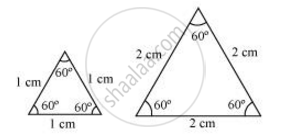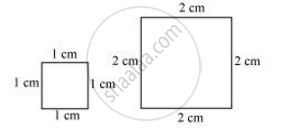# Give Two Different Examples of Pair of Similar Figures - Mathematics

Give two different examples of pair of similar figures

#### Solution

Two equilateral triangles with sides 1 cm and 2 cmTwo squares with sides 1 cm and 2 cmConcept: Similar Figures
Is there an error in this question or solution?
Chapter 6: Triangles - Exercise 6.1 [Page 122]

#### APPEARS IN

NCERT Class 10 Maths
Chapter 6 Triangles
Exercise 6.1 | Q 2.1 | Page 122

Share